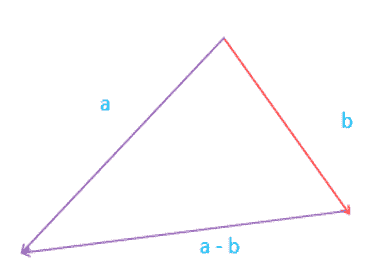# Calculate Difference of Two Dimensional Vectors

Calculate Difference of 2D Vectors for entered values for vector a and vector b:

 Vector a: , Vector b: .

 Subtraction: ;

Subtraction of vectors (difference of vectors) |A| ,|B| is operation of a finding of a vector |C|, coordinates of which are equal to a difference of all corresponding coordinates of vectors |A| & |B| can define as

ci = ai – bi.Vector subtraction is the simply negative of addition. Let's consider two vector a=[5,8] and b=[7,2].

So now we can say a - b = a + (-b).

Means that change the sign of b’s components like:

b=[7,2] will become as:

-b=[-7,-2]

Therefore a + (-b) = {5 + (-7)} , {8 + (-2)}

= (5 – 7) , (8 -2)

=(-2 , 6)